## Build a Neural Network

In this video we build our first image classifier using Keras and debug some of the common issues that come up.

Project

Check your understanding by building a fashion classifier by going to https://github.com/lukas/ml-class/tree/master/projects/1-fashion-mnist

Topics

• Line-by-line introduction to Keras
• Formatting image input for a neural network
• Weights
• Epochs
• Debugging models using activation functions

## Build a Neural Network

In this tutorial we are going to be using the canonical dataset MNIST, which contains images of handwritten digits. To run the code, follow the getting started instructions here. We will create a simple neural network, known as a perceptron, to classify these handwritten digits into ‘five’ or ‘not five’. This is known as optical character recognition.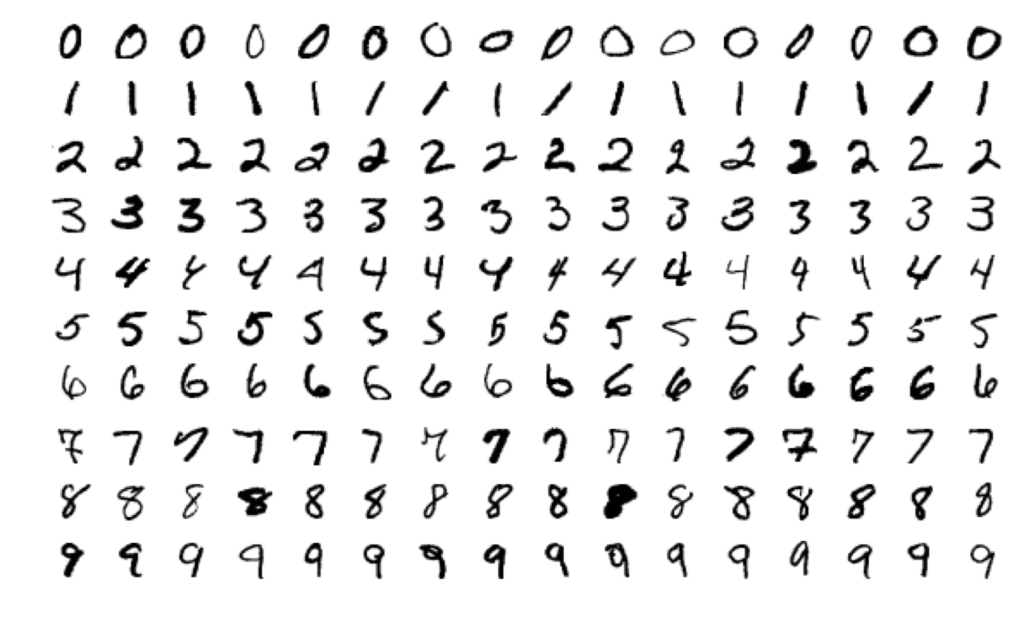MNIST: Modified National Institute of Standards and Technology

## Perceptrons

Perceptrons take in an array of numbers – in this case pixel values – and output a single number. The output will be a 1 if the digit is a five, and 0 if it is any other number. We train perceptrons with training data, which are the handwritten digits labelled with what number they are.

Perceptrons weight each of the input numbers to reach a correct classification. For instance, if we start with random weights and train our model with some data that it knows is a five, it will adjust how much it weights each of pixels depending on how predictive they are. This may mess up the weights for the earlier data but we don’t worry about that now.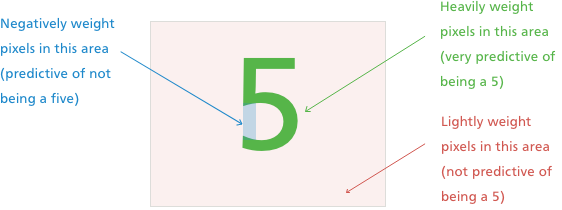Running through all of the data once and adjusting these weights is known as completing one epoch. After one epoch, we will have an initial guess at the weights for each pixel. We can then run through all of the data again and again, fine tuning the weights each time. Finally, we take the weighted sum of the pixel values to create an output number. We can interpret the output however we want: in this case anything greater than 0 means that it is a 5.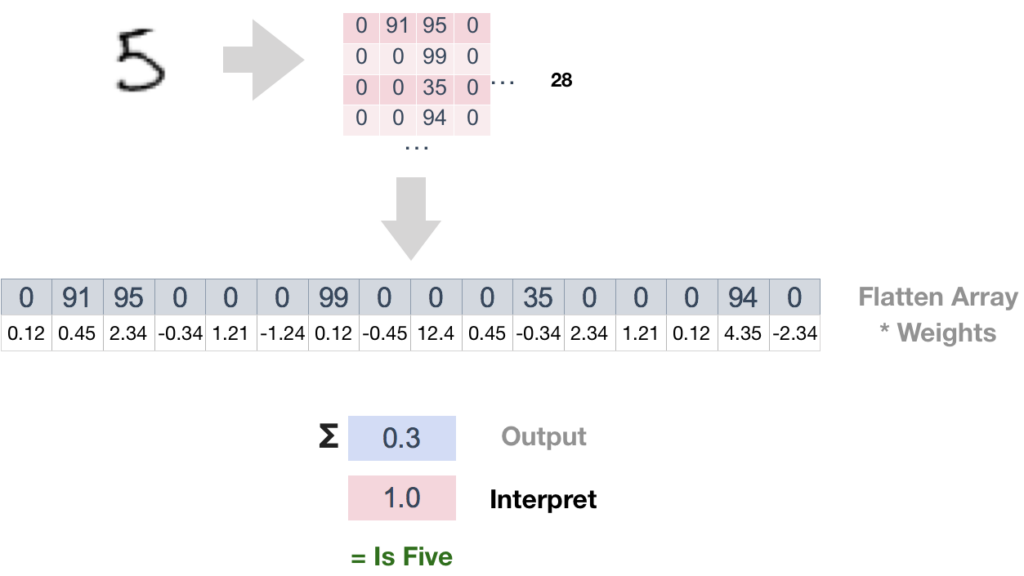## Understanding the code

How do we transfer our intuition to code? We use the popular Machine Learning library Keras to create our model in just 30 lines of code.We feed this model some training data, but hold out a portion as validation data. This is used to test our model later, to make sure that the model hasn’t just learnt the training data  (known as overfitting) and can generalize to new data too.

In order to find the model with the best weights, keras uses backpropagation. Backpropagation means that when the model adjusts the weights based on new inputs, it calculates how it would have affected the previous data that it has seen. This is the intuition for gradient descent.There are different ways to calculate loss, known as loss functions. In this section we use Mean Squared Error (MSE), which you can review in the first lecture.

Another parameter we define in our model is the learning rate. This determines how fast we adjust (or ‘learn’) weights in response to seeing new data. If this rate is too slow, it will take too long to learn the optimal weights. However, if the rate is too fast we may skip over the optimal weights. The best choice of learning rate depends on the exact problem, and is a focus of machine learning research.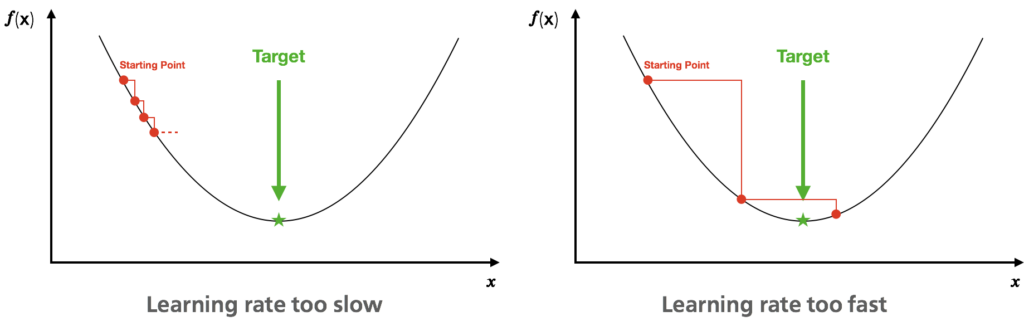To run this model, run python perceptron-single.py.

## Debugging

After running the neural network, you will notice that it has a very low accuracy, which doesn’t seem to improve with the number of epochs. How should we debug this model? If we print out our models output, we see that the model is outputting large negative and positive numbers: not numbers between 0 and 1 like we want.

Activation functions take the output from the model and turn them into the output that we want. In this case, we use a Sigmoidactivation function, which turns large positive numbers into 1, large negative numbers into 0, and everything in between into a value between 0 and 1. This ensures that the output is a number between 0 and 1, which we have told our perceptron to interpret as either a ‘five’ or ‘not five’.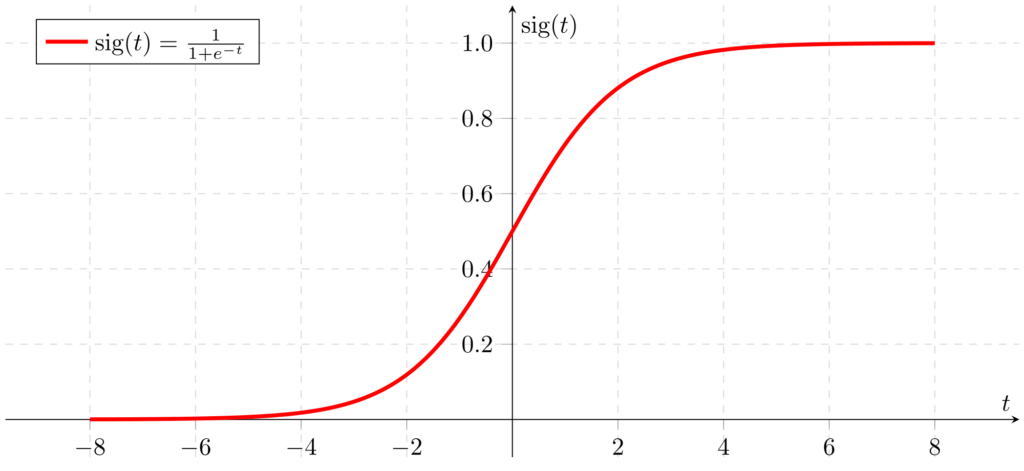We can add an activation function to our model on line 29 as follows: